#### Printable version

Detailed instructions for working a group puzzle of type 'HyperSudoku-by5'

The 9 elements in each group of this type of puzzle are: 1, 2, 3, 4, 5, 6, 7, 8, and 9.

Every group in the puzzle must contain exactly this set of elements.

####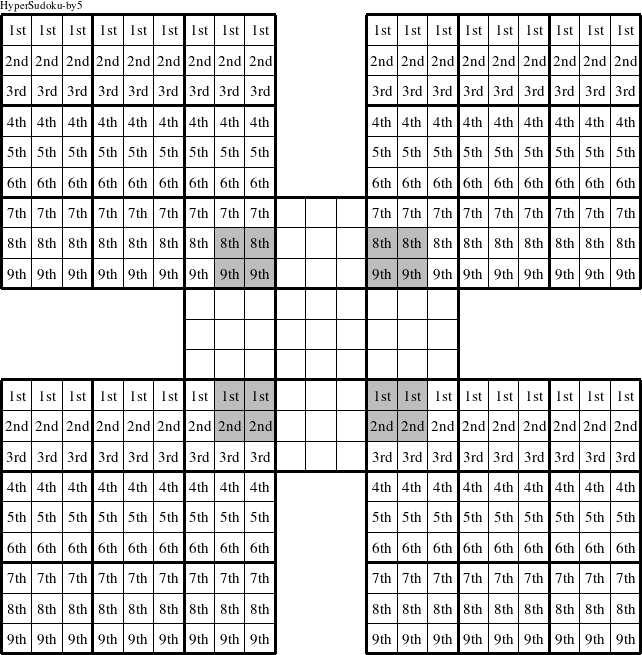In a HyperSudoku-by5 puzzle, each row in the upper left, upper right, lower left, and lower right puzzles is a group numbered as shown in the figure above.

####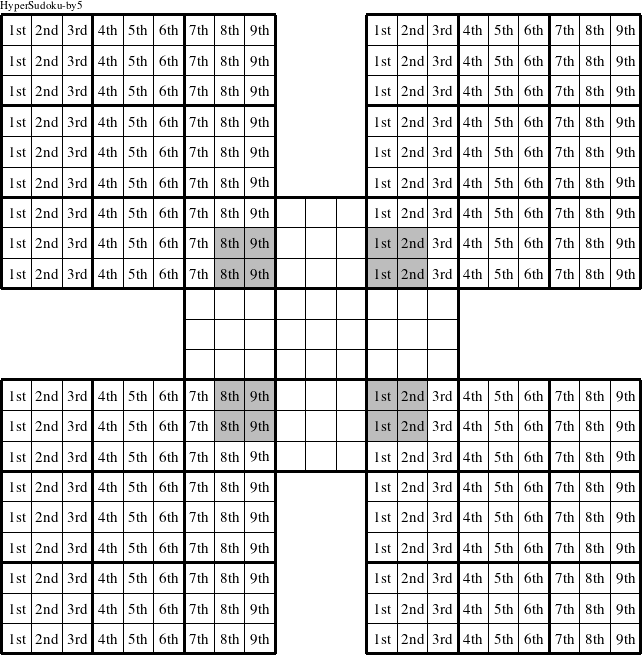In a HyperSudoku-by5 puzzle, each column in the upper left, upper right, lower left, and lower right puzzles is a group numbered as shown in the figure above.

####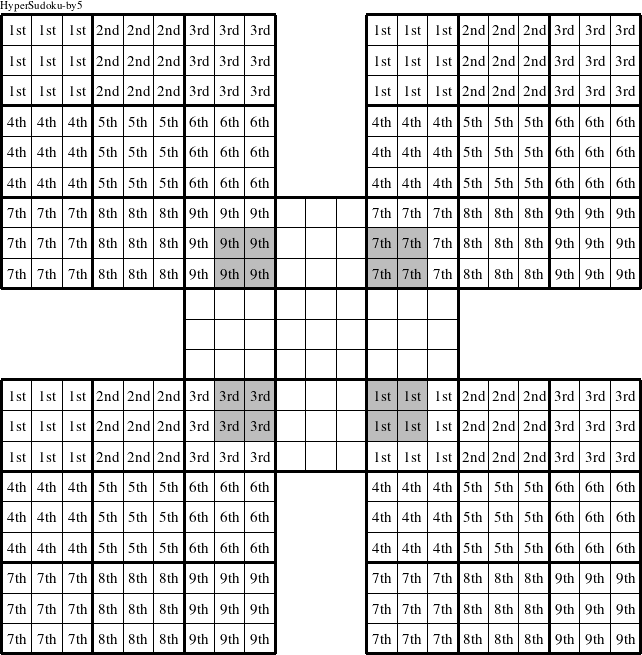In a HyperSudoku-by5 puzzle, each 3x3 square in the upper left, upper right, lower left, and lower right puzzles is a group numbered as shown in the figure above.

####In a HyperSudoku-by5 puzzle, each row in the center puzzle is a group numbered as shown in the figure above.

####In a HyperSudoku-by5 puzzle, each column in the center puzzle is a group numbered as shown in the figure above.

####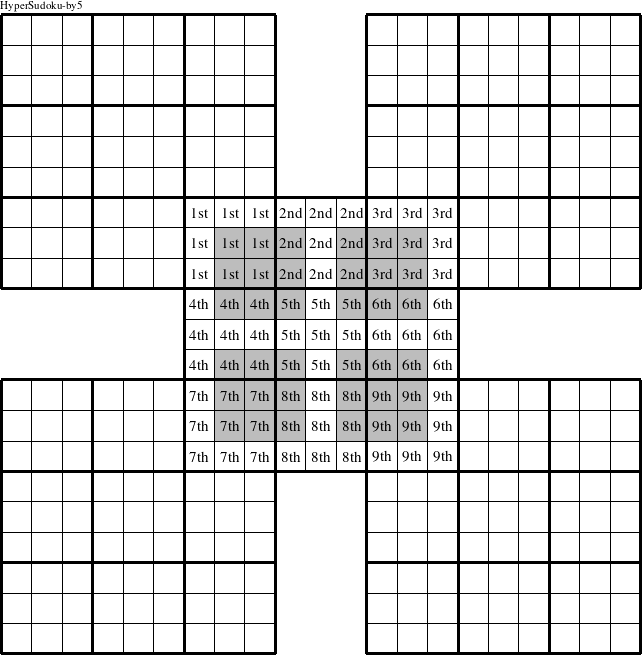In a HyperSudoku-by5 puzzle, each 3x3 square in the center puzzle is a group numbered as shown in the figure above.

####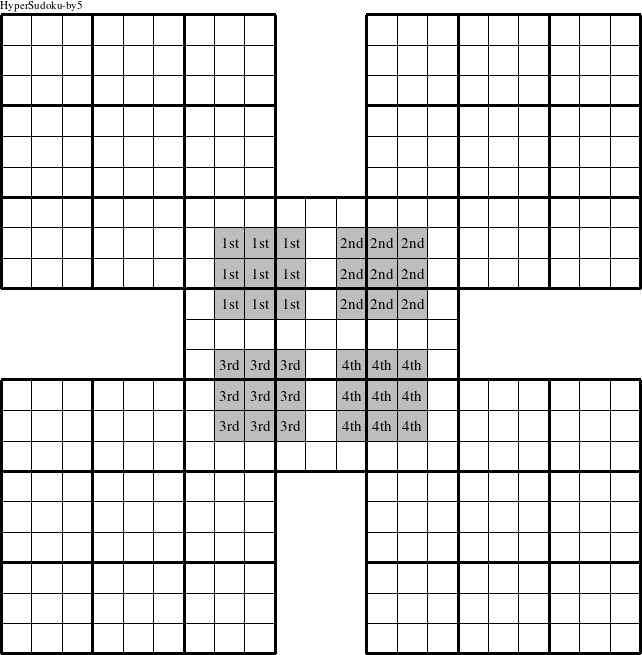In a HyperSudoku-by5 puzzle, each 3x3 inner square in the center puzzle is a group numbered as shown in the figure above.